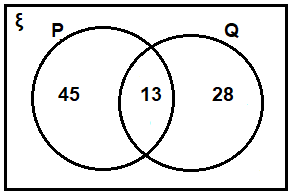# ML Aggarwal Solutions for Class 8 Maths Chapter 6 Operation On Sets Venn Diagram

ML Aggarwal Solutions for Class 8 Maths Chapter 6 Operation On Sets Venn Diagram PDF are very useful to help students understand the question pattern and type of different problems. It is essential to understand the various kinds of problems and figure out the best solutions for them. Referring to the ML Aggarwal Solutions for Class 8 Maths Chapter 6 can give a much better viewpoint and reference for understanding exercise.

Chapter 6 – Operation On Sets Venn Diagram. A set is a well-defined collection of distinct objects, considered as an object in its own right. For example, the numbers 1, 3, and 5 are distinct objects when considered separately, but when they are considered collectively they form a single set of size three, written {1, 3, 5}. Venn diagram is an illustration of the relationships between and among sets, groups of objects that share something in common. This ML Aggarwal Solutions for Class 8 Maths Chapter 6 contains the topics related to operations on sets and drawing of Venn diagrams.

## Download the PDF of ML Aggarwal Solutions for Class 8 Maths Chapter 6 Operation On Sets Venn Diagram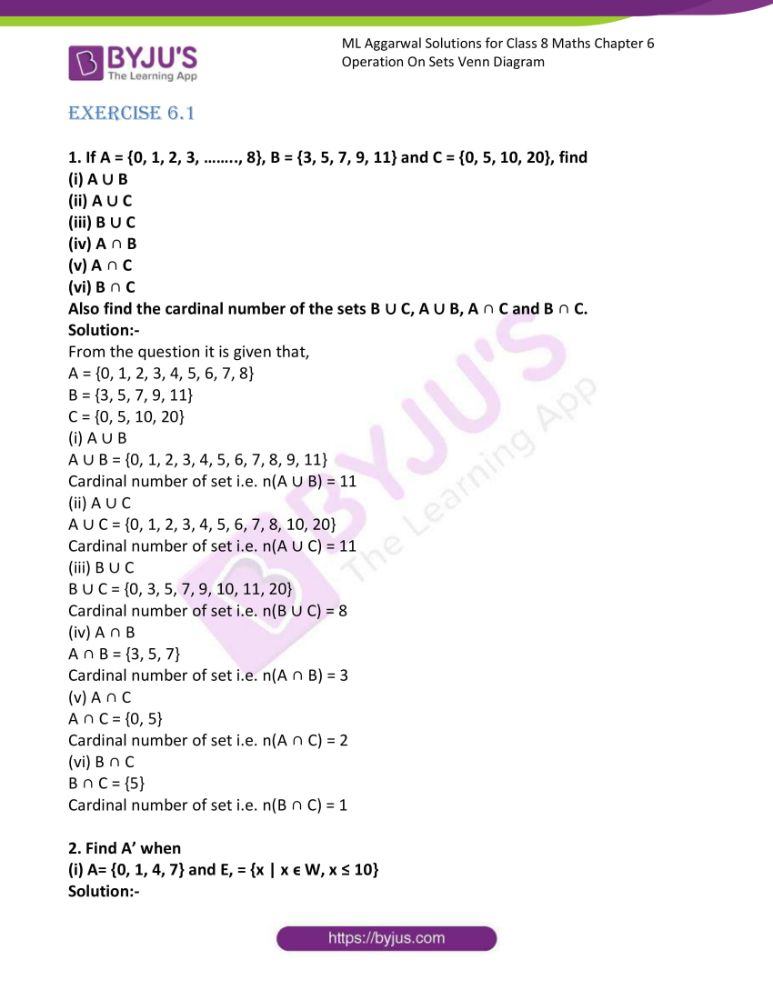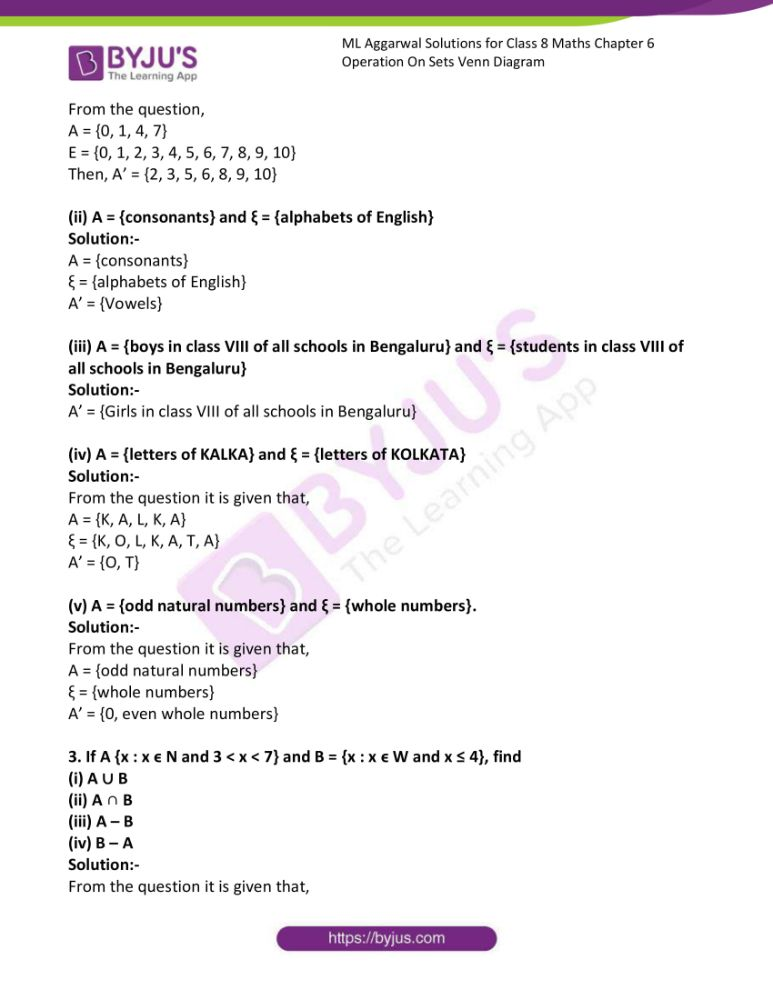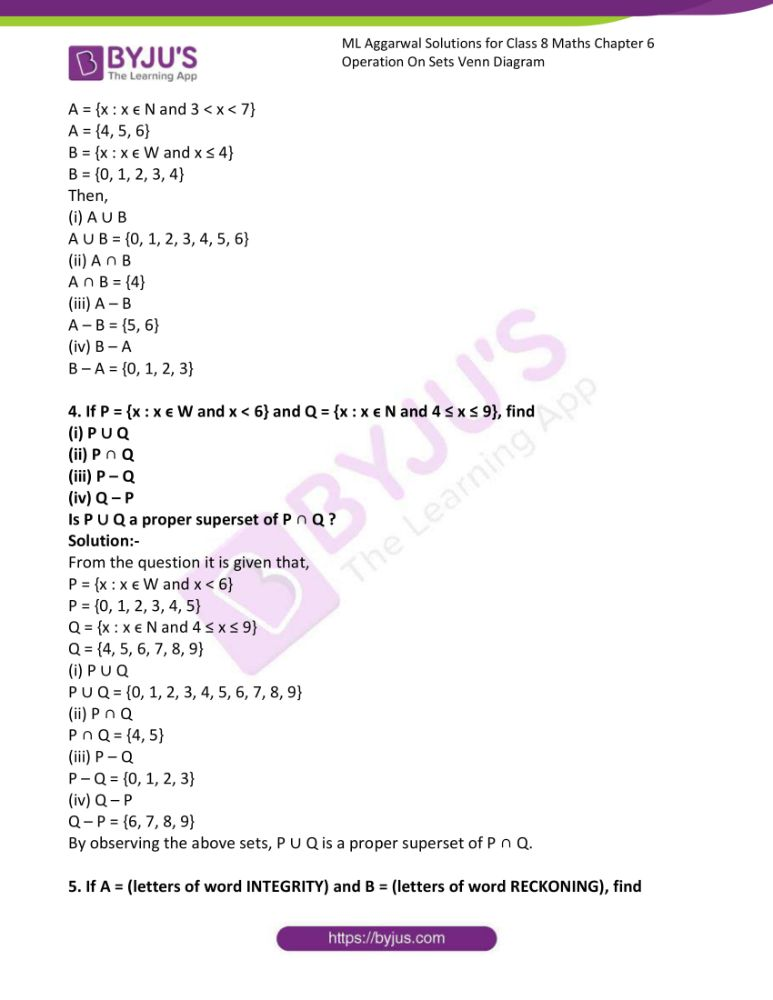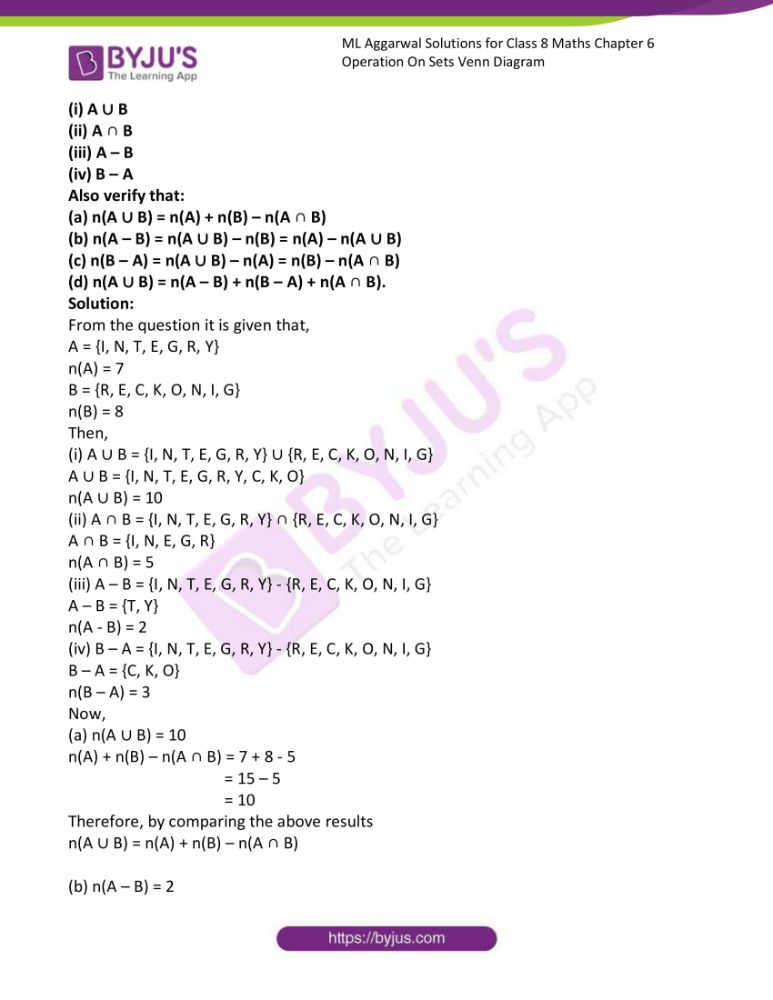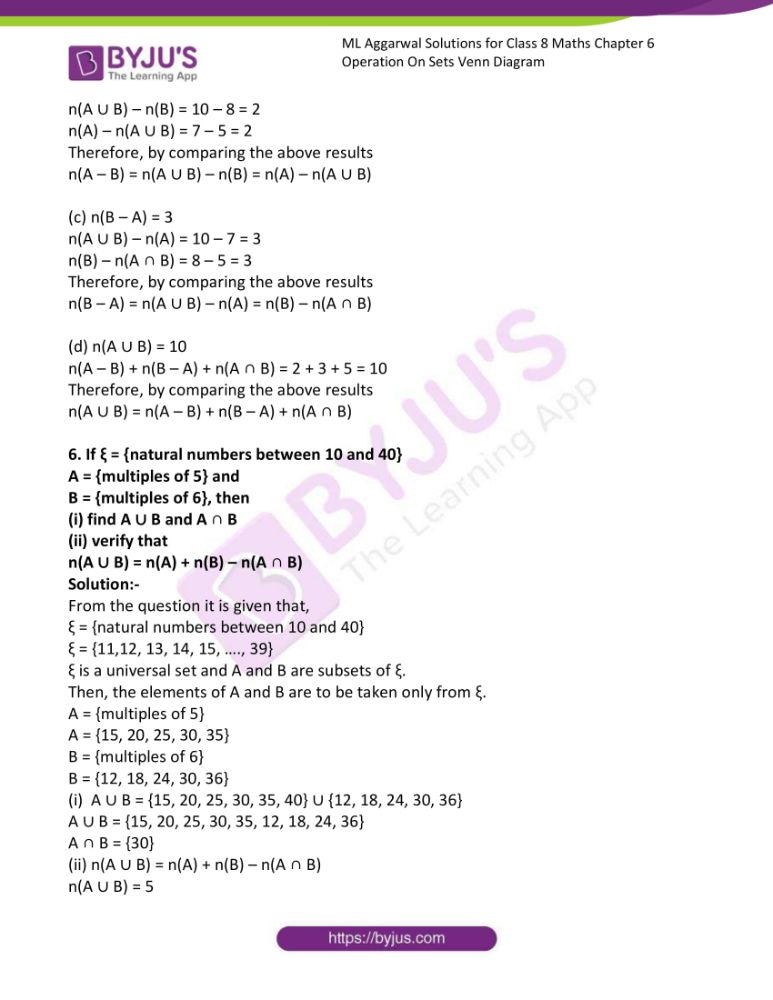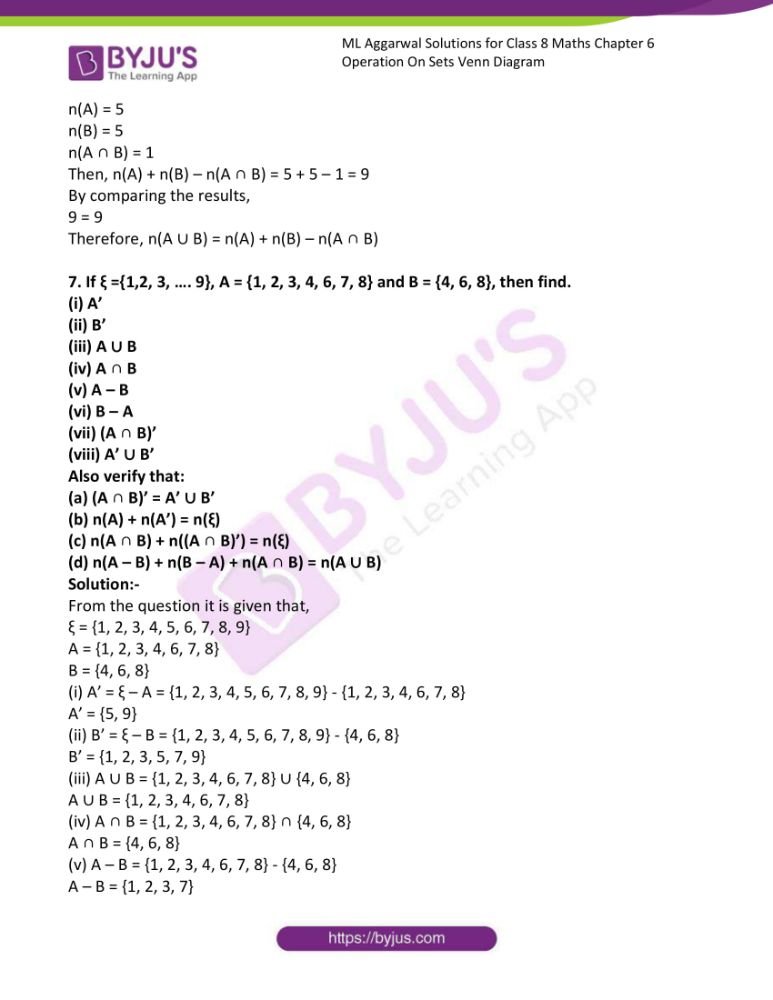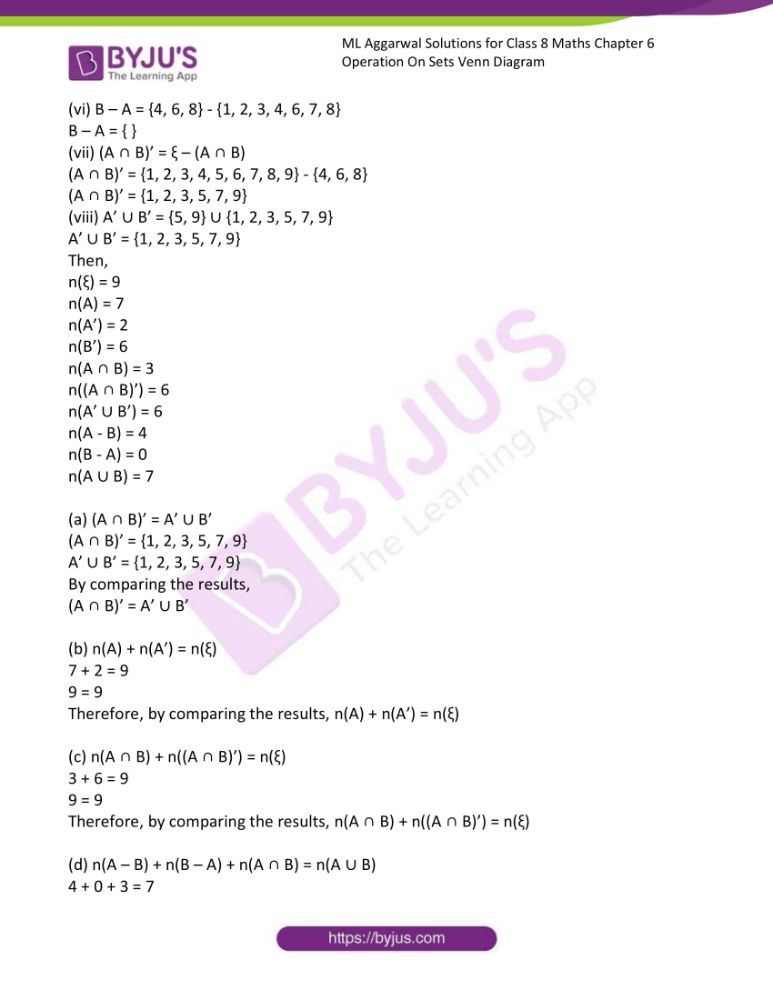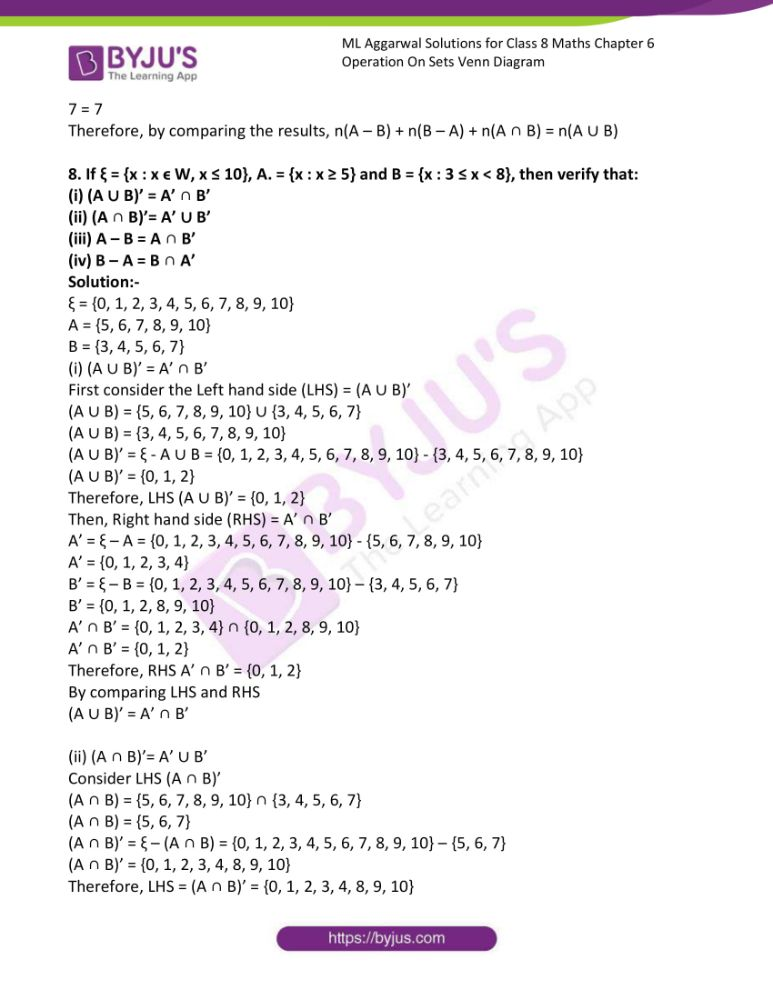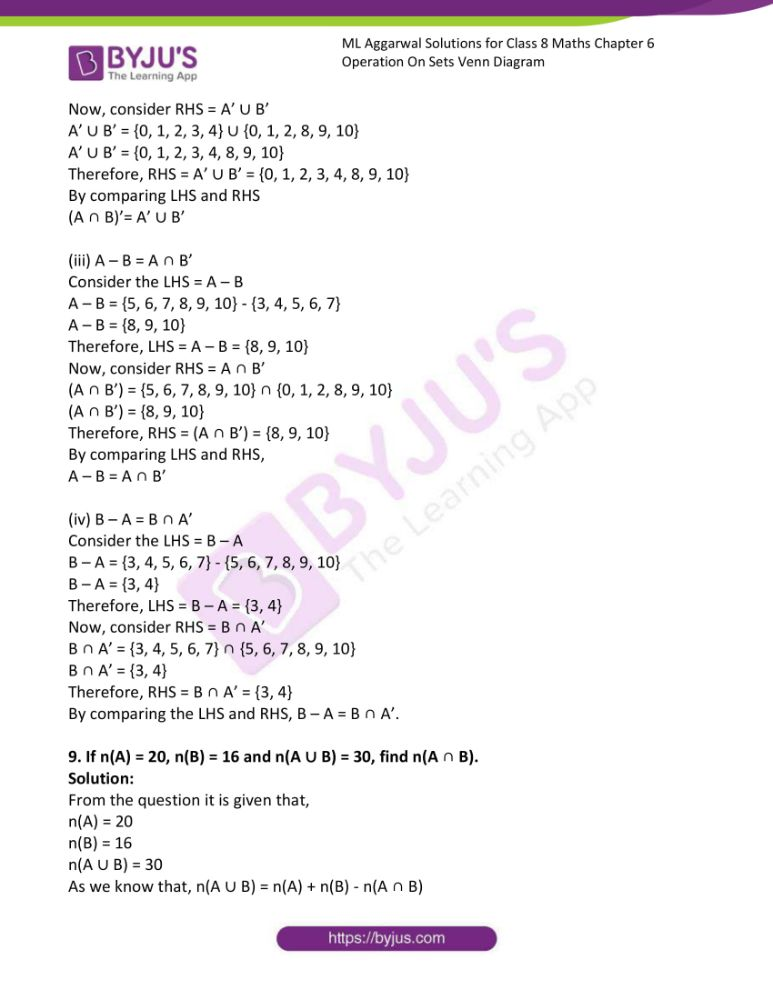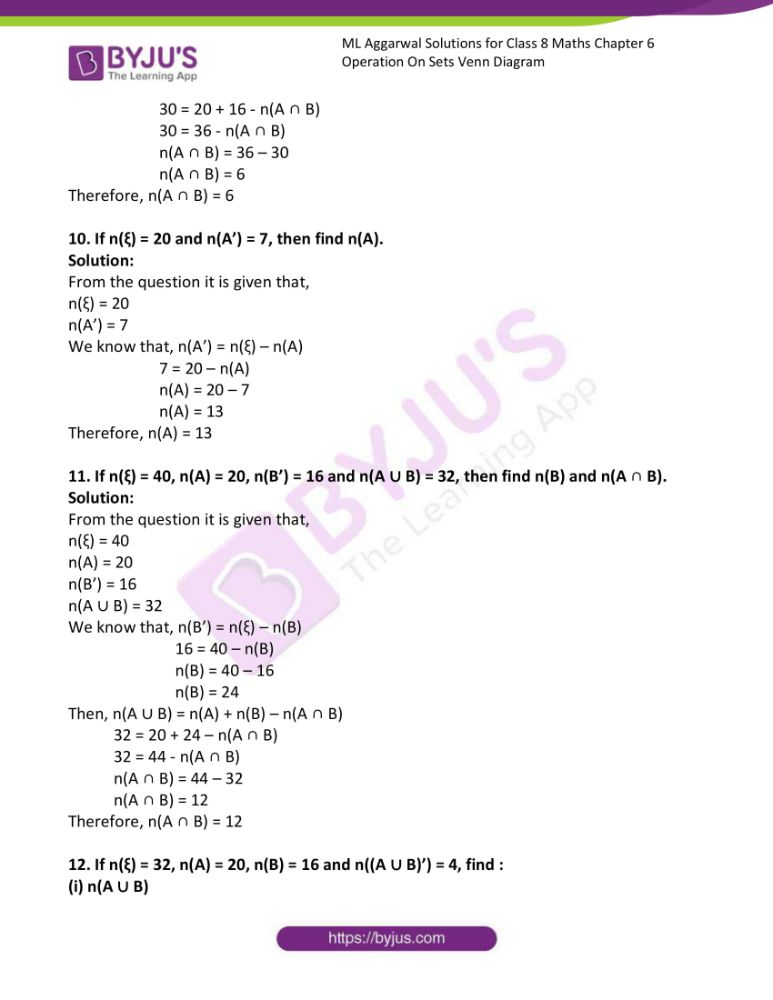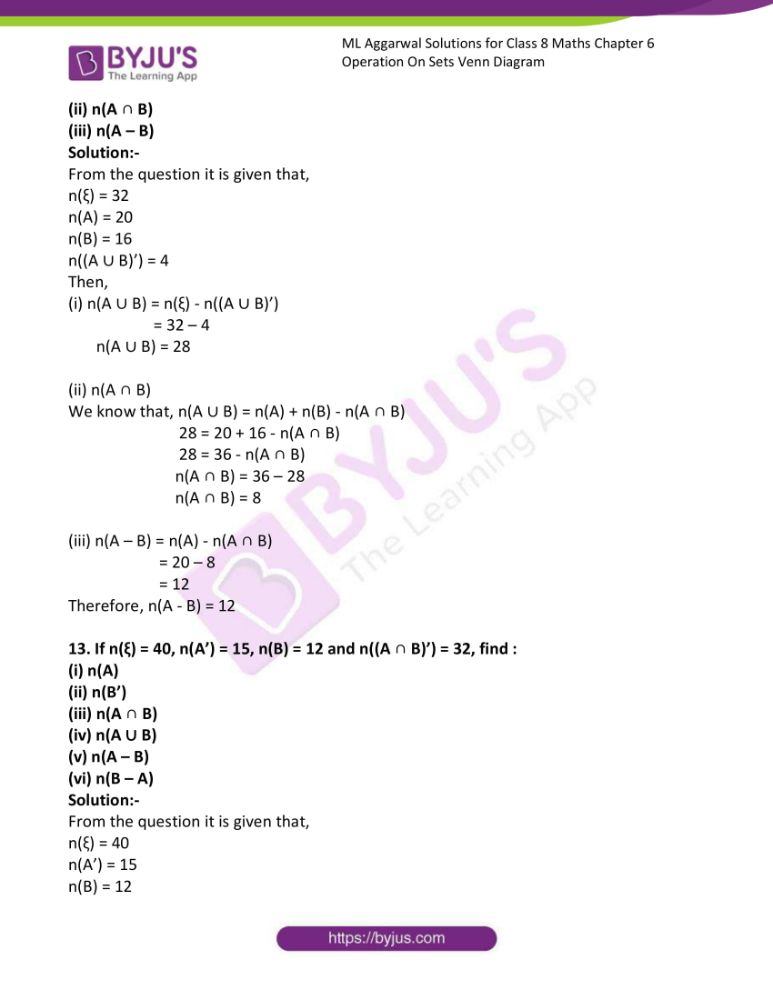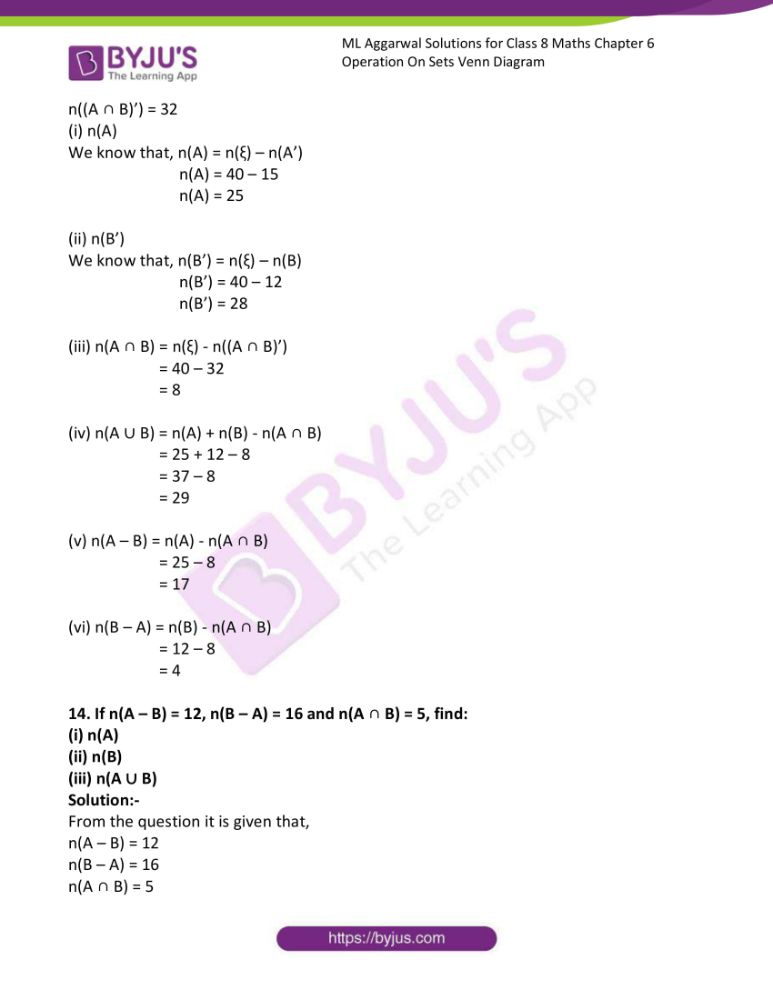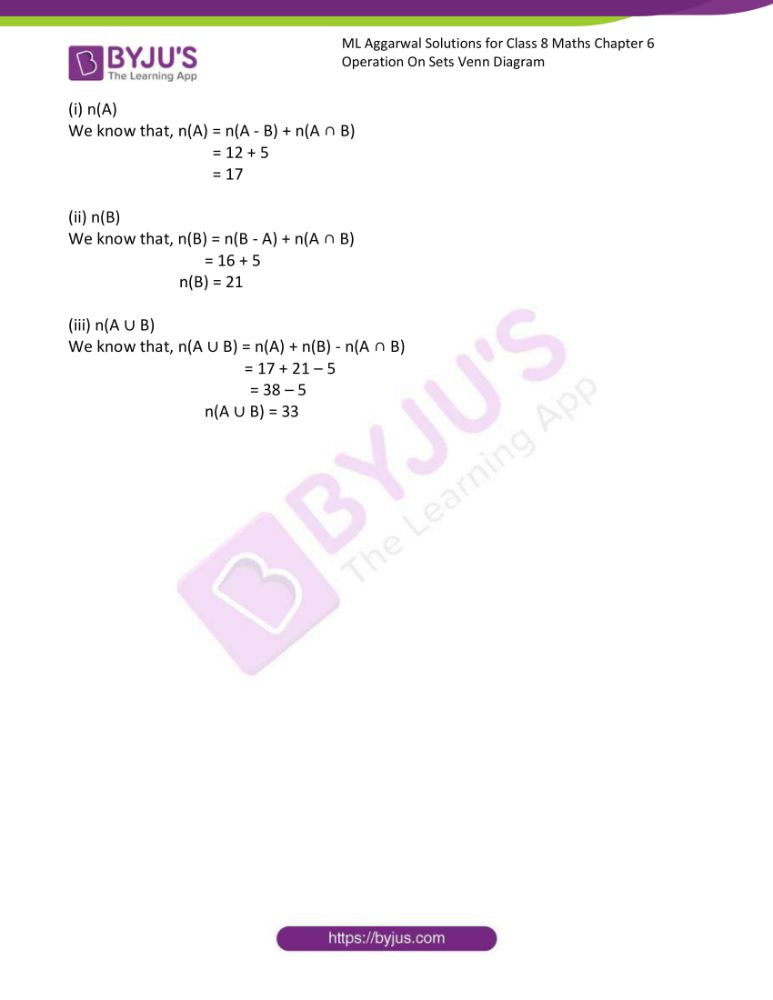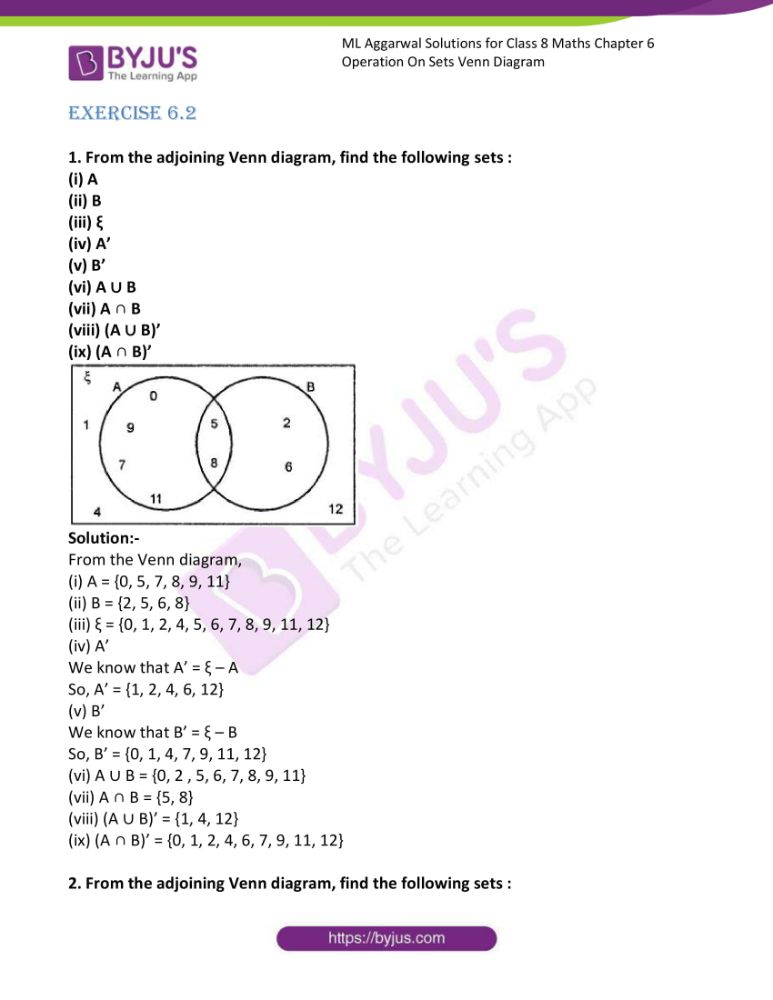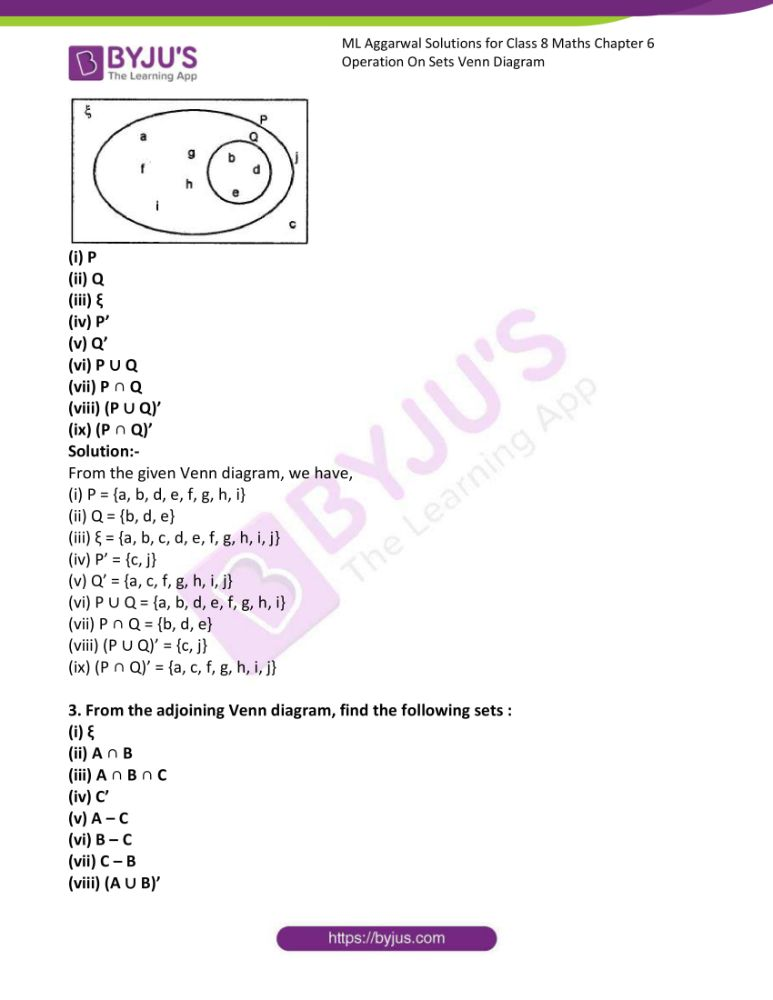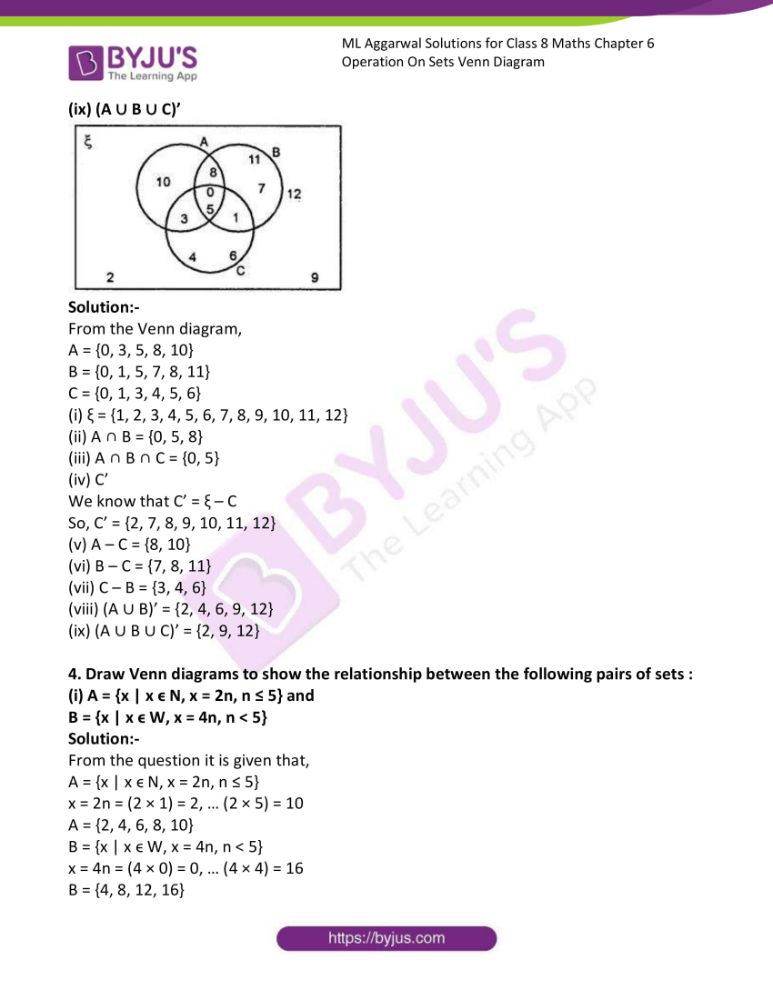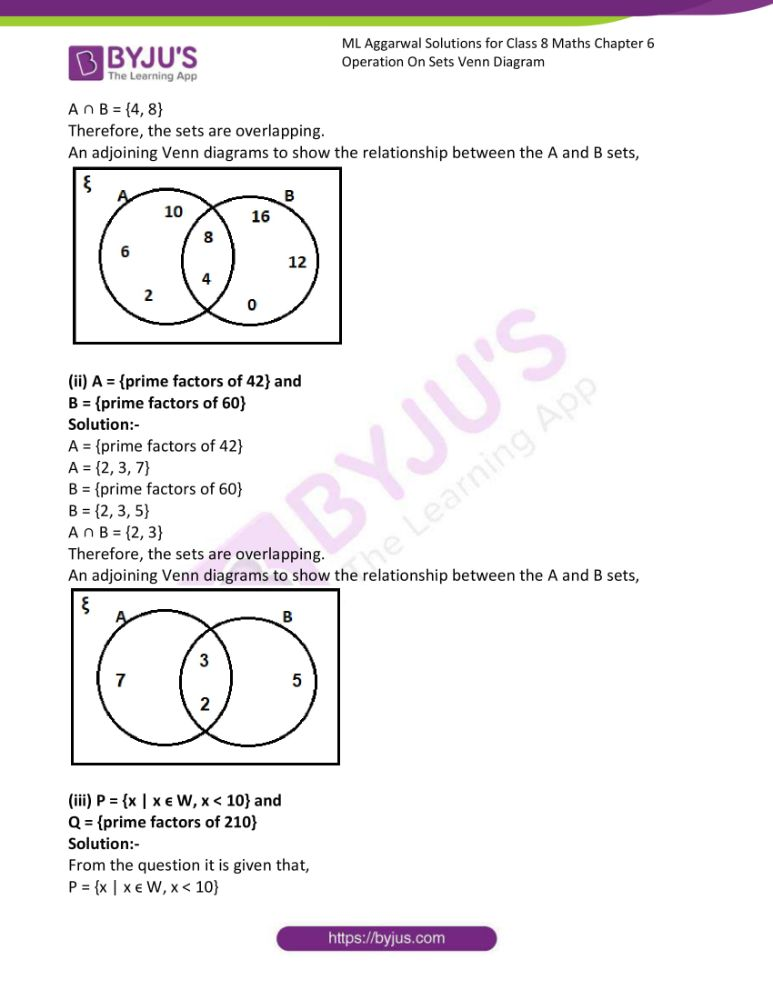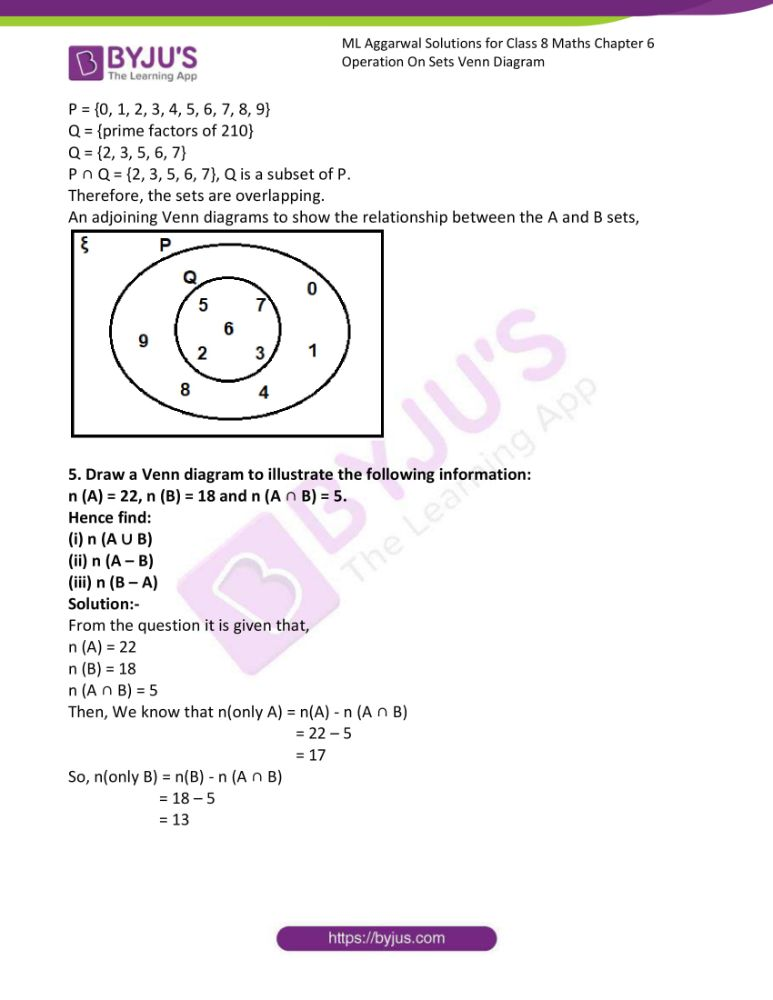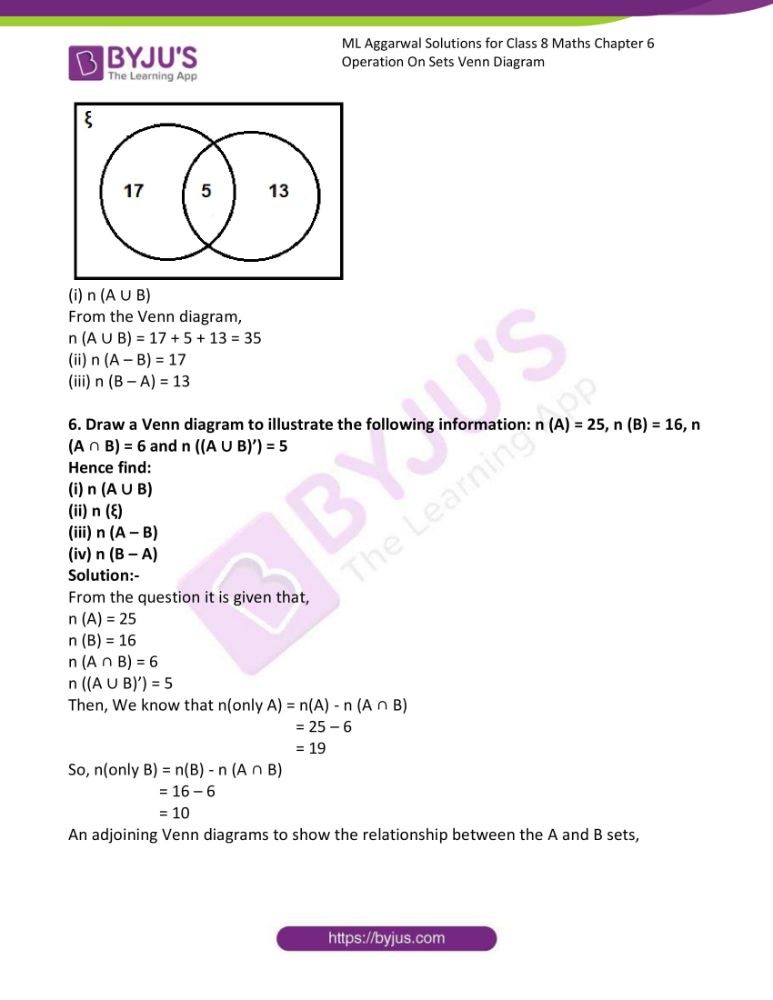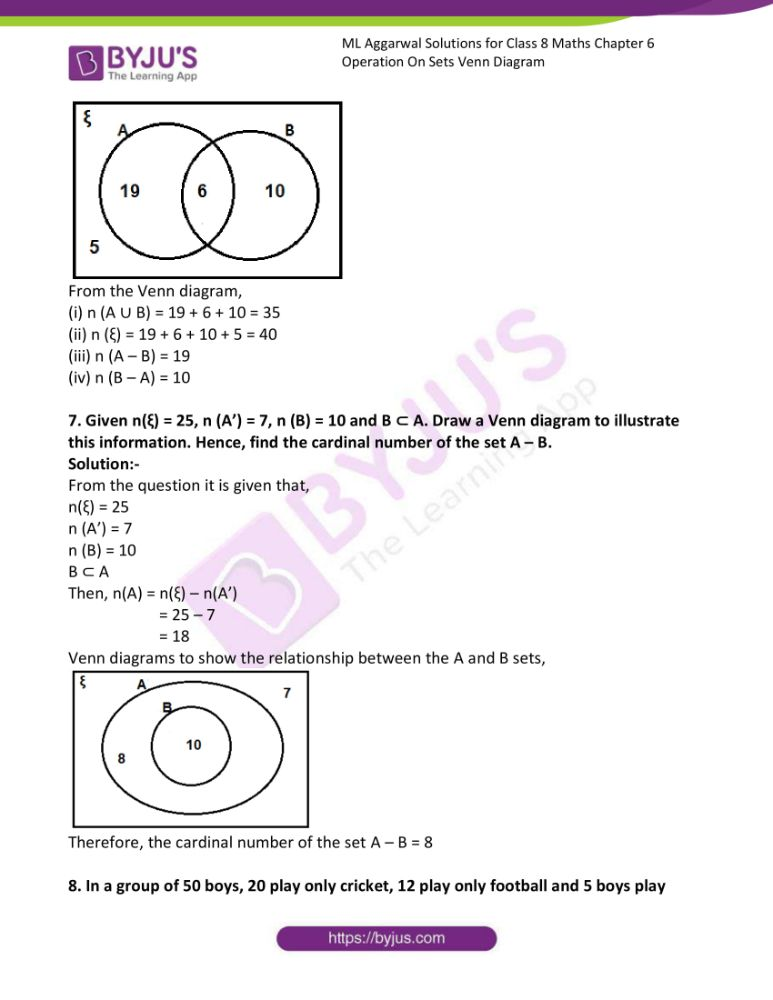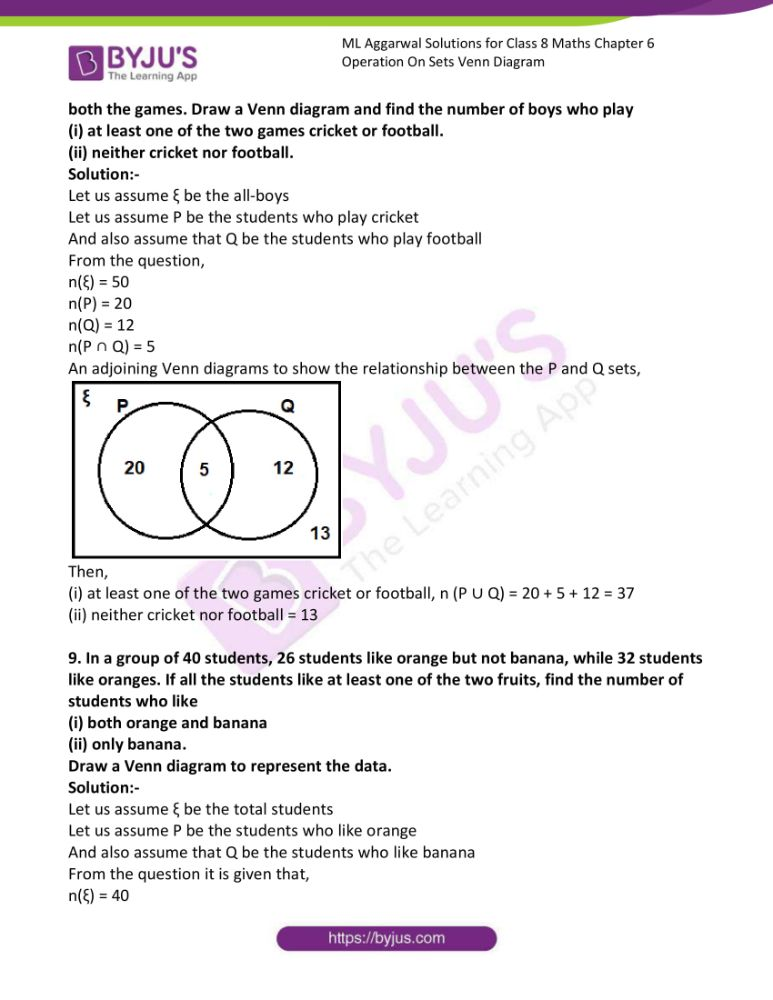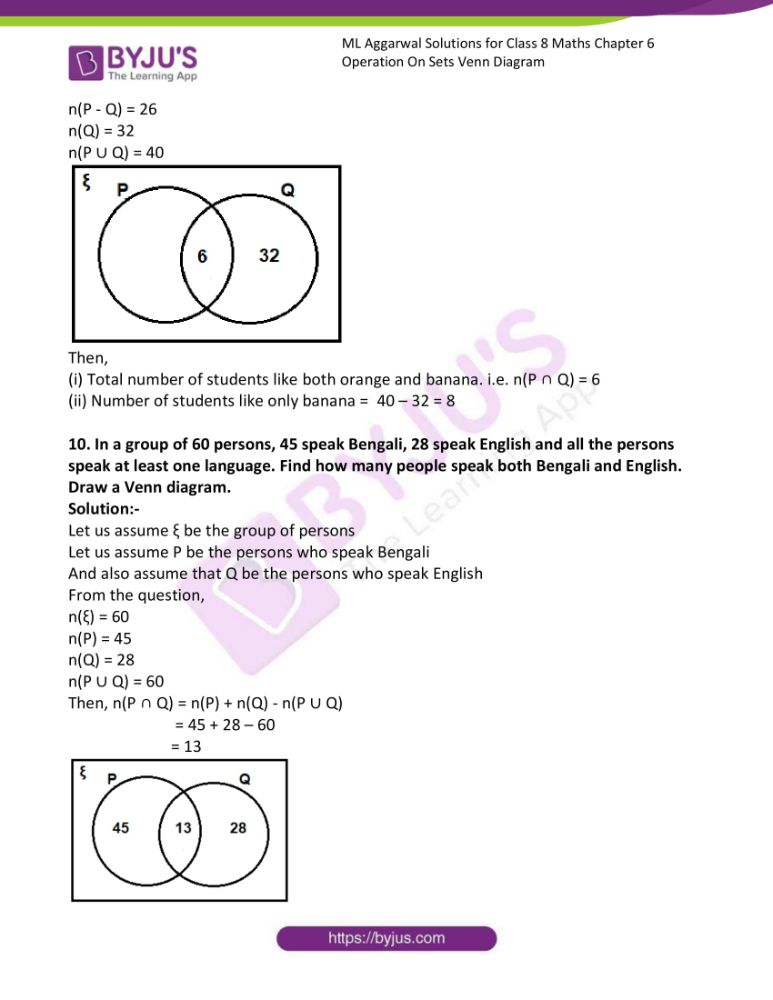### Access answers to ML Aggarwal Solutions for Class 8 Maths Chapter 6 Operation On Sets Venn Diagram

Exercise 6.1

1. If A = {0, 1, 2, 3, …….., 8}, B = {3, 5, 7, 9, 11} and C = {0, 5, 10, 20}, find
(i) A ∪ B
(ii) A ∪ C
(iii) B ∪ C
(iv) A ∩ B
(v) A ∩ C
(vi) B ∩ C
Also find the cardinal number of the sets B ∪ C, A ∪ B, A ∩ C and B ∩ C.

Solution:-

From the question it is given that,

A = {0, 1, 2, 3, 4, 5, 6, 7, 8}

B = {3, 5, 7, 9, 11}

C = {0, 5, 10, 20}

(i) A ∪ B

A ∪ B = {0, 1, 2, 3, 4, 5, 6, 7, 8, 9, 11}

Cardinal number of set i.e. n(A ∪ B) = 11

(ii) A ∪ C

A ∪ C = {0, 1, 2, 3, 4, 5, 6, 7, 8, 10, 20}

Cardinal number of set i.e. n(A ∪ C) = 11

(iii) B ∪ C

B ∪ C = {0, 3, 5, 7, 9, 10, 11, 20}

Cardinal number of set i.e. n(B ∪ C) = 8

(iv) A ∩ B

A ∩ B = {3, 5, 7}

Cardinal number of set i.e. n(A ∩ B) = 3

(v) A ∩ C

A ∩ C = {0, 5}

Cardinal number of set i.e. n(A ∩ C) = 2

(vi) B ∩ C

B ∩ C = {5}

Cardinal number of set i.e. n(B ∩ C) = 1

2. Find A’ when
(i) A= {0, 1, 4, 7} and E, = {x | x ϵ W, x ≤ 10}

Solution:-

From the question,

A = {0, 1, 4, 7}

E = {0, 1, 2, 3, 4, 5, 6, 7, 8, 9, 10}

Then, A’ = {2, 3, 5, 6, 8, 9, 10}

(ii) A = {consonants} and ξ = {alphabets of English}

Solution:-

A = {consonants}

ξ = {alphabets of English}

A’ = {Vowels}

(iii) A = {boys in class VIII of all schools in Bengaluru} and ξ = {students in class VIII of all schools in Bengaluru}

Solution:-

A’ = {Girls in class VIII of all schools in Bengaluru}

(iv) A = {letters of KALKA} and ξ = {letters of KOLKATA}

Solution:-

From the question it is given that,

A = {K, A, L, K, A}

ξ = {K, O, L, K, A, T, A}

A’ = {O, T}

(v) A = {odd natural numbers} and ξ = {whole numbers}.

Solution:-

From the question it is given that,

A = {odd natural numbers}

ξ = {whole numbers}

A’ = {0, even whole numbers}

3. If A {x : x ϵ N and 3 < x < 7} and B = {x : x ϵ W and x ≤ 4}, find
(i) A ∪ B
(ii) A ∩ B
(iii) A – B
(iv) B – A

Solution:-

From the question it is given that,

A = {x : x ϵ N and 3 < x < 7}

A = {4, 5, 6}

B = {x : x ϵ W and x ≤ 4}

B = {0, 1, 2, 3, 4}

Then,

(i) A ∪ B

A ∪ B = {0, 1, 2, 3, 4, 5, 6}

(ii) A ∩ B

A ∩ B = {4}

(iii) A – B

A – B = {5, 6}

(iv) B – A

B – A = {0, 1, 2, 3}

4. If P = {x : x ϵ W and x < 6} and Q = {x : x ϵ N and 4 ≤ x ≤ 9}, find
(i) P ∪ Q
(ii) P ∩ Q
(iii) P – Q
(iv) Q – P
Is P ∪ Q a proper superset of P ∩ Q ?

Solution:-

From the question it is given that,

P = {x : x ϵ W and x < 6}

P = {0, 1, 2, 3, 4, 5}

Q = {x : x ϵ N and 4 ≤ x ≤ 9}

Q = {4, 5, 6, 7, 8, 9}

(i) P ∪ Q

P ∪ Q = {0, 1, 2, 3, 4, 5, 6, 7, 8, 9}

(ii) P ∩ Q

P ∩ Q = {4, 5}

(iii) P – Q

P – Q = {0, 1, 2, 3}

(iv) Q – P

Q – P = {6, 7, 8, 9}

By observing the above sets, P ∪ Q is a proper superset of P ∩ Q.

5. If A = (letters of word INTEGRITY) and B = (letters of word RECKONING), find
(i) A ∪ B
(ii) A ∩ B
(iii) A – B
(iv) B – A
Also verify that:
(a) n(A ∪ B) = n(A) + n(B) – n(A ∩ B)
(b) n(A – B) = n(A ∪ B) – n(B) = n(A) – n(A ∪ B)
(c) n(B – A) = n(A ∪ B) – n(A) = n(B) – n(A ∩ B)
(d) n(A ∪ B) = n(A – B) + n(B – A) + n(A ∩ B).
Solution:

From the question it is given that,

A = {I, N, T, E, G, R, Y}

n(A) = 7

B = {R, E, C, K, O, N, I, G}

n(B) = 8

Then,

(i) A ∪ B = {I, N, T, E, G, R, Y} ∪ {R, E, C, K, O, N, I, G}

A ∪ B = {I, N, T, E, G, R, Y, C, K, O}

n(A ∪ B) = 10

(ii) A ∩ B = {I, N, T, E, G, R, Y} ∩ {R, E, C, K, O, N, I, G}

A ∩ B = {I, N, E, G, R}

n(A ∩ B) = 5

(iii) A – B = {I, N, T, E, G, R, Y} – {R, E, C, K, O, N, I, G}

A – B = {T, Y}

n(A – B) = 2

(iv) B – A = {I, N, T, E, G, R, Y} – {R, E, C, K, O, N, I, G}

B – A = {C, K, O}

n(B – A) = 3

Now,

(a) n(A ∪ B) = 10

n(A) + n(B) – n(A ∩ B) = 7 + 8 – 5

= 15 – 5

= 10

Therefore, by comparing the above results

n(A ∪ B) = n(A) + n(B) – n(A ∩ B)

(b) n(A – B) = 2

n(A ∪ B) – n(B) = 10 – 8 = 2

n(A) – n(A ∪ B) = 7 – 5 = 2

Therefore, by comparing the above results

n(A – B) = n(A ∪ B) – n(B) = n(A) – n(A ∪ B)

(c) n(B – A) = 3

n(A ∪ B) – n(A) = 10 – 7 = 3

n(B) – n(A ∩ B) = 8 – 5 = 3

Therefore, by comparing the above results

n(B – A) = n(A ∪ B) – n(A) = n(B) – n(A ∩ B)

(d) n(A ∪ B) = 10

n(A – B) + n(B – A) + n(A ∩ B) = 2 + 3 + 5 = 10

Therefore, by comparing the above results

n(A ∪ B) = n(A – B) + n(B – A) + n(A ∩ B)

6. If ξ = {natural numbers between 10 and 40}
A = {multiples of 5} and
B = {multiples of 6}, then
(i) find A ∪ B and A ∩ B
(ii) verify that
n(A ∪ B) = n(A) + n(B) – n(A ∩ B)

Solution:-

From the question it is given that,

ξ = {natural numbers between 10 and 40}

ξ = {11,12, 13, 14, 15, …., 39}

ξ is a universal set and A and B are subsets of ξ.

Then, the elements of A and B are to be taken only from ξ.

A = {multiples of 5}

A = {15, 20, 25, 30, 35}

B = {multiples of 6}

B = {12, 18, 24, 30, 36}

(i) A ∪ B = {15, 20, 25, 30, 35, 40} ∪ {12, 18, 24, 30, 36}

A ∪ B = {15, 20, 25, 30, 35, 12, 18, 24, 36}

A ∩ B = {30}

(ii) n(A ∪ B) = n(A) + n(B) – n(A ∩ B)

n(A ∪ B) = 5

n(A) = 5

n(B) = 5

n(A ∩ B) = 1

Then, n(A) + n(B) – n(A ∩ B) = 5 + 5 – 1 = 9

By comparing the results,

9 = 9

Therefore, n(A ∪ B) = n(A) + n(B) – n(A ∩ B)

7. If ξ ={1,2, 3, …. 9}, A = {1, 2, 3, 4, 6, 7, 8} and B = {4, 6, 8}, then find.
(i) A’
(ii) B’
(iii) A ∪ B
(iv) A ∩ B
(v) A – B
(vi) B – A
(vii) (A ∩ B)’
(viii) A’ ∪ B’
Also verify that:
(a) (A ∩ B)’ = A’ ∪ B’
(b) n(A) + n(A’) = n(ξ)
(c) n(A ∩ B) + n((A ∩ B)’) = n(ξ)
(d) n(A – B) + n(B – A) + n(A ∩ B) = n(A ∪ B)

Solution:-

From the question it is given that,

ξ = {1, 2, 3, 4, 5, 6, 7, 8, 9}

A = {1, 2, 3, 4, 6, 7, 8}

B = {4, 6, 8}

(i) A’ = ξ – A = {1, 2, 3, 4, 5, 6, 7, 8, 9} – {1, 2, 3, 4, 6, 7, 8}

A’ = {5, 9}

(ii) B’ = ξ – B = {1, 2, 3, 4, 5, 6, 7, 8, 9} – {4, 6, 8}

B’ = {1, 2, 3, 5, 7, 9}

(iii) A ∪ B = {1, 2, 3, 4, 6, 7, 8} ∪ {4, 6, 8}

A ∪ B = {1, 2, 3, 4, 6, 7, 8}

(iv) A ∩ B = {1, 2, 3, 4, 6, 7, 8} ∩ {4, 6, 8}

A ∩ B = {4, 6, 8}

(v) A – B = {1, 2, 3, 4, 6, 7, 8} – {4, 6, 8}

A – B = {1, 2, 3, 7}

(vi) B – A = {4, 6, 8} – {1, 2, 3, 4, 6, 7, 8}

B – A = { }

(vii) (A ∩ B)’ = ξ – (A ∩ B)

(A ∩ B)’ = {1, 2, 3, 4, 5, 6, 7, 8, 9} – {4, 6, 8}

(A ∩ B)’ = {1, 2, 3, 5, 7, 9}

(viii) A’ ∪ B’ = {5, 9} ∪ {1, 2, 3, 5, 7, 9}

A’ ∪ B’ = {1, 2, 3, 5, 7, 9}

Then,

n(ξ) = 9

n(A) = 7

n(A’) = 2

n(B’) = 6

n(A ∩ B) = 3

n((A ∩ B)’) = 6

n(A’ ∪ B’) = 6

n(A – B) = 4

n(B – A) = 0

n(A ∪ B) = 7

(a) (A ∩ B)’ = A’ ∪ B’

(A ∩ B)’ = {1, 2, 3, 5, 7, 9}

A’ ∪ B’ = {1, 2, 3, 5, 7, 9}

By comparing the results,

(A ∩ B)’ = A’ ∪ B’

(b) n(A) + n(A’) = n(ξ)

7 + 2 = 9

9 = 9

Therefore, by comparing the results, n(A) + n(A’) = n(ξ)

(c) n(A ∩ B) + n((A ∩ B)’) = n(ξ)

3 + 6 = 9

9 = 9

Therefore, by comparing the results, n(A ∩ B) + n((A ∩ B)’) = n(ξ)

(d) n(A – B) + n(B – A) + n(A ∩ B) = n(A ∪ B)

4 + 0 + 3 = 7

7 = 7

Therefore, by comparing the results, n(A – B) + n(B – A) + n(A ∩ B) = n(A ∪ B)

8. If ξ = {x : x ϵ W, x ≤ 10}, A. = {x : x ≥ 5} and B = {x : 3 ≤ x < 8}, then verify that:
(i) (A ∪ B)’ = A’ ∩ B’
(ii) (A ∩ B)’= A’ ∪ B’
(iii) A – B = A ∩ B’
(iv) B – A = B ∩ A’

Solution:-

ξ = {0, 1, 2, 3, 4, 5, 6, 7, 8, 9, 10}

A = {5, 6, 7, 8, 9, 10}

B = {3, 4, 5, 6, 7}

(i) (A ∪ B)’ = A’ ∩ B’

First consider the Left hand side (LHS) = (A ∪ B)’

(A ∪ B) = {5, 6, 7, 8, 9, 10} ∪ {3, 4, 5, 6, 7}

(A ∪ B) = {3, 4, 5, 6, 7, 8, 9, 10}

(A ∪ B)’ = ξ – A ∪ B = {0, 1, 2, 3, 4, 5, 6, 7, 8, 9, 10} – {3, 4, 5, 6, 7, 8, 9, 10}

(A ∪ B)’ = {0, 1, 2}

Therefore, LHS (A ∪ B)’ = {0, 1, 2}

Then, Right hand side (RHS) = A’ ∩ B’

A’ = ξ – A = {0, 1, 2, 3, 4, 5, 6, 7, 8, 9, 10} – {5, 6, 7, 8, 9, 10}

A’ = {0, 1, 2, 3, 4}

B’ = ξ – B = {0, 1, 2, 3, 4, 5, 6, 7, 8, 9, 10} – {3, 4, 5, 6, 7}

B’ = {0, 1, 2, 8, 9, 10}

A’ ∩ B’ = {0, 1, 2, 3, 4} ∩ {0, 1, 2, 8, 9, 10}

A’ ∩ B’ = {0, 1, 2}

Therefore, RHS A’ ∩ B’ = {0, 1, 2}

By comparing LHS and RHS

(A ∪ B)’ = A’ ∩ B’

(ii) (A ∩ B)’= A’ ∪ B’

Consider LHS (A ∩ B)’

(A ∩ B) = {5, 6, 7, 8, 9, 10} ∩ {3, 4, 5, 6, 7}

(A ∩ B) = {5, 6, 7}

(A ∩ B)’ = ξ – (A ∩ B) = {0, 1, 2, 3, 4, 5, 6, 7, 8, 9, 10} – {5, 6, 7}

(A ∩ B)’ = {0, 1, 2, 3, 4, 8, 9, 10}

Therefore, LHS = (A ∩ B)’ = {0, 1, 2, 3, 4, 8, 9, 10}

Now, consider RHS = A’ ∪ B’

A’ ∪ B’ = {0, 1, 2, 3, 4} ∪ {0, 1, 2, 8, 9, 10}

A’ ∪ B’ = {0, 1, 2, 3, 4, 8, 9, 10}

Therefore, RHS = A’ ∪ B’ = {0, 1, 2, 3, 4, 8, 9, 10}

By comparing LHS and RHS

(A ∩ B)’= A’ ∪ B’

(iii) A – B = A ∩ B’

Consider the LHS = A – B

A – B = {5, 6, 7, 8, 9, 10} – {3, 4, 5, 6, 7}

A – B = {8, 9, 10}

Therefore, LHS = A – B = {8, 9, 10}

Now, consider RHS = A ∩ B’

(A ∩ B’) = {5, 6, 7, 8, 9, 10} ∩ {0, 1, 2, 8, 9, 10}

(A ∩ B’) = {8, 9, 10}

Therefore, RHS = (A ∩ B’) = {8, 9, 10}

By comparing LHS and RHS,

A – B = A ∩ B’

(iv) B – A = B ∩ A’

Consider the LHS = B – A

B – A = {3, 4, 5, 6, 7} – {5, 6, 7, 8, 9, 10}

B – A = {3, 4}

Therefore, LHS = B – A = {3, 4}

Now, consider RHS = B ∩ A’

B ∩ A’ = {3, 4, 5, 6, 7} ∩ {5, 6, 7, 8, 9, 10}

B ∩ A’ = {3, 4}

Therefore, RHS = B ∩ A’ = {3, 4}

By comparing the LHS and RHS, B – A = B ∩ A’.

9. If n(A) = 20, n(B) = 16 and n(A ∪ B) = 30, find n(A ∩ B).
Solution:

From the question it is given that,

n(A) = 20

n(B) = 16

n(A ∪ B) = 30

As we know that, n(A ∪ B) = n(A) + n(B) – n(A ∩ B)

30 = 20 + 16 – n(A ∩ B)

30 = 36 – n(A ∩ B)

n(A ∩ B) = 36 – 30

n(A ∩ B) = 6

Therefore, n(A ∩ B) = 6

10. If n(ξ) = 20 and n(A’) = 7, then find n(A).
Solution:

From the question it is given that,

n(ξ) = 20

n(A’) = 7

We know that, n(A’) = n(ξ) – n(A)

7 = 20 – n(A)

n(A) = 20 – 7

n(A) = 13

Therefore, n(A) = 13

11. If n(ξ) = 40, n(A) = 20, n(B’) = 16 and n(A ∪ B) = 32, then find n(B) and n(A ∩ B).
Solution:

From the question it is given that,

n(ξ) = 40

n(A) = 20

n(B’) = 16

n(A ∪ B) = 32

We know that, n(B’) = n(ξ) – n(B)

16 = 40 – n(B)

n(B) = 40 – 16

n(B) = 24

Then, n(A ∪ B) = n(A) + n(B) – n(A ∩ B)

32 = 20 + 24 – n(A ∩ B)

32 = 44 – n(A ∩ B)

n(A ∩ B) = 44 – 32

n(A ∩ B) = 12

Therefore, n(A ∩ B) = 12

12. If n(ξ) = 32, n(A) = 20, n(B) = 16 and n((A ∪ B)’) = 4, find :
(i) n(A ∪ B)
(ii) n(A ∩ B)
(iii) n(A – B)

Solution:-

From the question it is given that,

n(ξ) = 32

n(A) = 20

n(B) = 16

n((A ∪ B)’) = 4

Then,

(i) n(A ∪ B) = n(ξ) – n((A ∪ B)’)

= 32 – 4

n(A ∪ B) = 28

(ii) n(A ∩ B)

We know that, n(A ∪ B) = n(A) + n(B) – n(A ∩ B)

28 = 20 + 16 – n(A ∩ B)

28 = 36 – n(A ∩ B)

n(A ∩ B) = 36 – 28

n(A ∩ B) = 8

(iii) n(A – B) = n(A) – n(A ∩ B)

= 20 – 8

= 12

Therefore, n(A – B) = 12

13. If n(ξ) = 40, n(A’) = 15, n(B) = 12 and n((A ∩ B)’) = 32, find :
(i) n(A)
(ii) n(B’)
(iii) n(A ∩ B)
(iv) n(A ∪ B)
(v) n(A – B)
(vi) n(B – A)

Solution:-

From the question it is given that,

n(ξ) = 40

n(A’) = 15

n(B) = 12

n((A ∩ B)’) = 32

(i) n(A)

We know that, n(A) = n(ξ) – n(A’)

n(A) = 40 – 15

n(A) = 25

(ii) n(B’)

We know that, n(B’) = n(ξ) – n(B)

n(B’) = 40 – 12

n(B’) = 28

(iii) n(A ∩ B) = n(ξ) – n((A ∩ B)’)

= 40 – 32

= 8

(iv) n(A ∪ B) = n(A) + n(B) – n(A ∩ B)

= 25 + 12 – 8

= 37 – 8

= 29

(v) n(A – B) = n(A) – n(A ∩ B)

= 25 – 8

= 17

(vi) n(B – A) = n(B) – n(A ∩ B)

= 12 – 8

= 4

14. If n(A – B) = 12, n(B – A) = 16 and n(A ∩ B) = 5, find:
(i) n(A)
(ii) n(B)
(iii) n(A ∪ B)

Solution:-

From the question it is given that,

n(A – B) = 12

n(B – A) = 16

n(A ∩ B) = 5

(i) n(A)

We know that, n(A) = n(A – B) + n(A ∩ B)

= 12 + 5

= 17

(ii) n(B)

We know that, n(B) = n(B – A) + n(A ∩ B)

= 16 + 5

n(B) = 21

(iii) n(A ∪ B)

We know that, n(A ∪ B) = n(A) + n(B) – n(A ∩ B)

= 17 + 21 – 5

= 38 – 5

n(A ∪ B) = 33

Exercise 6.2

1. From the adjoining Venn diagram, find the following sets :
(i) A
(ii) B
(iii) ξ
(iv) A’
(v) B’
(vi) A ∪ B
(vii) A ∩ B
(viii) (A ∪ B)’
(ix) (A ∩ B)’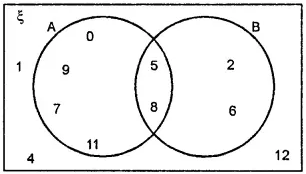Solution:-

From the Venn diagram,

(i) A = {0, 5, 7, 8, 9, 11}

(ii) B = {2, 5, 6, 8}

(iii) ξ = {0, 1, 2, 4, 5, 6, 7, 8, 9, 11, 12}

(iv) A’

We know that A’ = ξ – A

So, A’ = {1, 2, 4, 6, 12}

(v) B’

We know that B’ = ξ – B

So, B’ = {0, 1, 4, 7, 9, 11, 12}

(vi) A ∪ B = {0, 2 , 5, 6, 7, 8, 9, 11}

(vii) A ∩ B = {5, 8}

(viii) (A ∪ B)’ = {1, 4, 12}

(ix) (A ∩ B)’ = {0, 1, 2, 4, 6, 7, 9, 11, 12}

2. From the adjoining Venn diagram, find the following sets :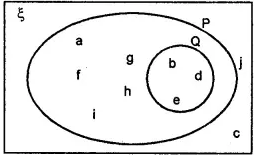(i) P
(ii) Q
(iii) ξ
(iv) P’
(v) Q’
(vi) P ∪ Q
(vii) P ∩ Q
(viii) (P ∪ Q)’
(ix) (P ∩ Q)’

Solution:-

From the given Venn diagram, we have,

(i) P = {a, b, d, e, f, g, h, i}

(ii) Q = {b, d, e}

(iii) ξ = {a, b, c, d, e, f, g, h, i, j}

(iv) P’ = {c, j}

(v) Q’ = {a, c, f, g, h, i, j}

(vi) P ∪ Q = {a, b, d, e, f, g, h, i}

(vii) P ∩ Q = {b, d, e}

(viii) (P ∪ Q)’ = {c, j}

(ix) (P ∩ Q)’ = {a, c, f, g, h, i, j}

3. From the adjoining Venn diagram, find the following sets :
(i) ξ
(ii) A ∩ B
(iii) A ∩ B ∩ C
(iv) C’
(v) A – C
(vi) B – C
(vii) C – B
(viii) (A ∪ B)’
(ix) (A ∪ B ∪ C)’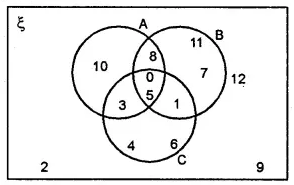Solution:-

From the Venn diagram,

A = {0, 3, 5, 8, 10}

B = {0, 1, 5, 7, 8, 11}

C = {0, 1, 3, 4, 5, 6}

(i) ξ = {1, 2, 3, 4, 5, 6, 7, 8, 9, 10, 11, 12}

(ii) A ∩ B = {0, 5, 8}

(iii) A ∩ B ∩ C = {0, 5}

(iv) C’

We know that C’ = ξ – C

So, C’ = {2, 7, 8, 9, 10, 11, 12}

(v) A – C = {8, 10}

(vi) B – C = {7, 8, 11}

(vii) C – B = {3, 4, 6}

(viii) (A ∪ B)’ = {2, 4, 6, 9, 12}

(ix) (A ∪ B ∪ C)’ = {2, 9, 12}

4. Draw Venn diagrams to show the relationship between the following pairs of sets :
(i) A = {x | x ϵ N, x = 2n, n ≤ 5} and
B = {x | x ϵ W, x = 4n, n < 5}

Solution:-

From the question it is given that,

A = {x | x ϵ N, x = 2n, n ≤ 5}

x = 2n = (2 × 1) = 2, … (2 × 5) = 10

A = {2, 4, 6, 8, 10}

B = {x | x ϵ W, x = 4n, n < 5}

x = 4n = (4 × 0) = 0, … (4 × 4) = 16

B = {4, 8, 12, 16}

A ∩ B = {4, 8}

Therefore, the sets are overlapping.

An adjoining Venn diagrams to show the relationship between the A and B sets,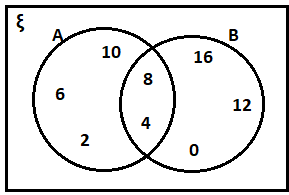(ii) A = {prime factors of 42} and
B = {prime factors of 60}

Solution:-

A = {prime factors of 42}

A = {2, 3, 7}

B = {prime factors of 60}

B = {2, 3, 5}

A ∩ B = {2, 3}

Therefore, the sets are overlapping.

An adjoining Venn diagrams to show the relationship between the A and B sets,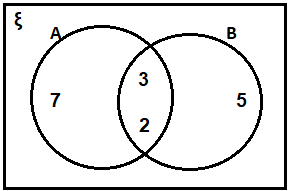(iii) P = {x | x ϵ W, x < 10} and
Q = {prime factors of 210}

Solution:-

From the question it is given that,

P = {x | x ϵ W, x < 10}

P = {0, 1, 2, 3, 4, 5, 6, 7, 8, 9}

Q = {prime factors of 210}

Q = {2, 3, 5, 6, 7}

P ∩ Q = {2, 3, 5, 6, 7}, Q is a subset of P.

Therefore, the sets are overlapping.

An adjoining Venn diagrams to show the relationship between the A and B sets,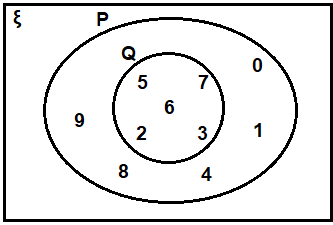5. Draw a Venn diagram to illustrate the following information:
n (A) = 22, n (B) = 18 and n (A ∩ B) = 5.
Hence find:
(i) n (A ∪ B)
(ii) n (A – B)
(iii) n (B – A)

Solution:-

From the question it is given that,

n (A) = 22

n (B) = 18

n (A ∩ B) = 5

Then, We know that n(only A) = n(A) – n (A ∩ B)

= 22 – 5

= 17

So, n(only B) = n(B) – n (A ∩ B)

= 18 – 5

= 13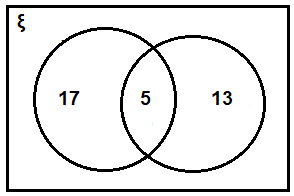(i) n (A ∪ B)

From the Venn diagram,

n (A ∪ B) = 17 + 5 + 13 = 35

(ii) n (A – B) = 17

(iii) n (B – A) = 13

6. Draw a Venn diagram to illustrate the following information: n (A) = 25, n (B) = 16, n (A ∩ B) = 6 and n ((A ∪ B)’) = 5
Hence find:
(i) n (A ∪ B)
(ii) n (ξ)
(iii) n (A – B)
(iv) n (B – A)

Solution:-

From the question it is given that,

n (A) = 25

n (B) = 16

n (A ∩ B) = 6

n ((A ∪ B)’) = 5

Then, We know that n(only A) = n(A) – n (A ∩ B)

= 25 – 6

= 19

So, n(only B) = n(B) – n (A ∩ B)

= 16 – 6

= 10

An adjoining Venn diagrams to show the relationship between the A and B sets,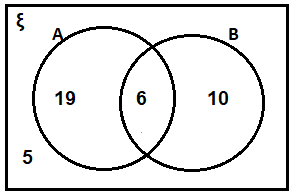From the Venn diagram,

(i) n (A ∪ B) = 19 + 6 + 10 = 35

(ii) n (ξ) = 19 + 6 + 10 + 5 = 40

(iii) n (A – B) = 19

(iv) n (B – A) = 10

7. Given n(ξ) = 25, n (A’) = 7, n (B) = 10 and B ⊂ A. Draw a Venn diagram to illustrate this information. Hence, find the cardinal number of the set A – B.

Solution:-

From the question it is given that,

n(ξ) = 25

n (A’) = 7

n (B) = 10

B ⊂ A

Then, n(A) = n(ξ) – n(A’)

= 25 – 7

= 18

Venn diagrams to show the relationship between the A and B sets,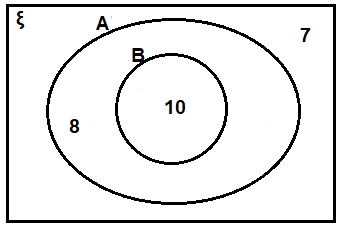Therefore, the cardinal number of the set A – B = 8

8. In a group of 50 boys, 20 play only cricket, 12 play only football and 5 boys play both the games. Draw a Venn diagram and find the number of boys who play
(i) at least one of the two games cricket or football.
(ii) neither cricket nor football.

Solution:-

Let us assume ξ be the all-boys

Let us assume P be the students who play cricket

And also assume that Q be the students who play football

From the question,

n(ξ) = 50

n(P) = 20

n(Q) = 12

n(P ∩ Q) = 5

An adjoining Venn diagrams to show the relationship between the P and Q sets,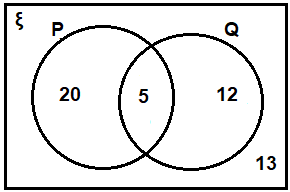Then,

(i) at least one of the two games cricket or football, n (P ∪ Q) = 20 + 5 + 12 = 37

(ii) neither cricket nor football = 13

9. In a group of 40 students, 26 students like orange but not banana, while 32 students like oranges. If all the students like at least one of the two fruits, find the number of students who like
(i) both orange and banana
(ii) only banana.
Draw a Venn diagram to represent the data.

Solution:-

Let us assume ξ be the total students

Let us assume P be the students who like orange

And also assume that Q be the students who like banana

From the question it is given that,

n(ξ) = 40

n(P – Q) = 26

n(Q) = 32

n(P ∪ Q) = 40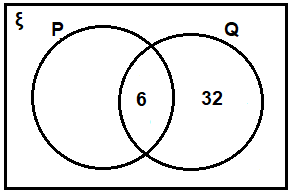Then,

(i) Total number of students like both orange and banana. i.e. n(P ∩ Q) = 6

(ii) Number of students like only banana = 40 – 32 = 8

10. In a group of 60 persons, 45 speak Bengali, 28 speak English and all the persons speak at least one language. Find how many people speak both Bengali and English. Draw a Venn diagram.

Solution:-

Let us assume ξ be the group of persons

Let us assume P be the persons who speak Bengali

And also assume that Q be the persons who speak English

From the question,

n(ξ) = 60

n(P) = 45

n(Q) = 28

n(P ∪ Q) = 60

Then, n(P ∩ Q) = n(P) + n(Q) – n(P ∪ Q)

= 45 + 28 – 60

= 13